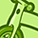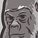Announcements
MAINTENANCE ALERT: Dec. 12th starting 9 AM CET. Community will be read-only. GET DETAILS
cancel
Showing results for
Did you mean:Not applicable

## Count using Set analysis in chart expression

Hi Experts,

I want to get the count of distinct encounterid where the paid amount of that encounterid is less then or equal to zero.

I tried the following expressions i cant get the result

=Count({<EncounterID ={"=\$(=Sum(PaidAmount)<=0)"}>}DISTINCT EncounterID)--Zero value is return

=Count({<EncounterID={"=(Sum(PaidAmount))<=0"}>} DISTINCT EncounterID)--Some wrong value is returned

i use the dimension as monthyear.

Can any one help me to come out of this problem

Regards,

S.Amuthabharathi

7 RepliesMVP

I assume you want your set expression to consider your chart dimension, right? This will not work, please check

Evaluating "sets" in the context of a dimensionCreator III

Try with Aggr function as below:

If(Aggr(Sum(PaidAmount),EncounterID) <= 0, count(DISTINCT EncounterID))

Regards,

SomNot applicable
Author

=Count({<PaidAmount={"=\$(=Sum(PaidAmount)<=0)"}>}DISTINCT EncounterID)Employee

Try

Count({<EncounterID={"=Sum(PaidAmount)<=0"}>} DISTINCT EncounterID)Not applicable
Author

Hi

i tried this too but i can get  the answer only -Not applicable
Author

I know this post has been open for a while - but I had the same issue and finally got it to work with the below expression. Perhaps it can help the next user

=COUNT(DISTINCT if(aggr(sum(PaidAmount),EncounterID) <= 0, EncounterID))Contributor II

This construct also worked for me.

I had to compare a date field to my master calendar like so:

=COUNT(DISTINCT if(Einddatum >= MonthEnd(Caldatum), Number))

But is is super slow, so I need something else. Any idea's?

For every new problem someone probably found a solution already ...Community Browser